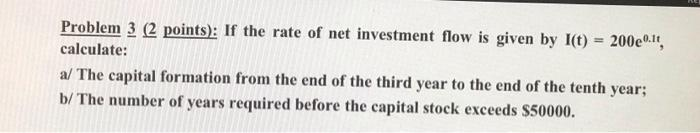# Question help me with this Problem 3 (2 points): If the rate of net investment flow is given by l(t) = 200e.lt, calculate: a/ The capital formation from the end of the third year to the end of the tenth year; b/ The number of years required before the capital stock exceeds $50000.1PWXZ8 The Asker · Other Mathematics help me with thisTranscribed Image Text: Problem 3 (2 points): If the rate of net investment flow is given by l(t) = 200e.lt, calculate: a/ The capital formation from the end of the third year to the end of the tenth year; b/ The number of years required before the capital stock exceeds$50000.
More
Transcribed Image Text: Problem 3 (2 points): If the rate of net investment flow is given by l(t) = 200e.lt, calculate: a/ The capital formation from the end of the third year to the end of the tenth year; b/ The number of years required before the capital stock exceeds \$50000.